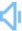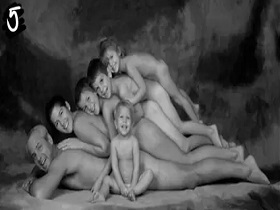Directly to word page Vague search(google)

## Graph in a sentence

Sentence count:213+4 Only show simple sentencesPosted:2016-12-24Updated:2020-07-24
Synonym: plotSimilar words: Meaning: [græf /grɑːf]n. a visual representation of the relations between certain quantities plotted with reference to a set of axes. v. 1. represent by means of a graph 2. plot upon a graph.Random good picture Not show
1. The statistics can be depicted as a graph.
2. They made a graph of weather.
3. Line the pages for the graph both horizontally and vertically.
4. This graph shows how crime has varied in relationship to unemployment over the last 20 years.
5. Plot a graph of height against age.
6. Keith was drawing a complicated-looking graph.
7. We plot about eight points on the graph.
8. The graph flattens out gradually after a steep fall.
9. Martin showed me a graph of their recent sales.
10. The figures are all plotted on a graph.
11. Results can be shown in numeric or graph form.
12. The graph showed peaks and troughs of activity.
13. First, plot the temperature curve on the graph.
14. We plotted a graph to show the increase in sales figures this year.
15. From this graph, it can be seen that some people are more susceptible to the disease.
16. The unemployment-income curve on the graph has a negative slope.
17. She drew a graph showing the relationship between costs and sales.
17. Wish you can benefit from sentencedict.com and make progress everyday!
18. The graph shows how house prices have risen since the 1980s.
19. We can see on this graph how the company has grown over the last year.
20. The intersection of the lines on the graph marks the point where we start to make a profit.
21. It is clear from the graph that sales have dropped sharply.
22. Sales targets are indicated on the graph by a vertical dotted line.
23. We need some sort of graph on which we can chart our progress.
24. They made a graph of how hot the weather was every day for a month.
25. The importance of the connexion graph is emphasised.
26. Our soundings are displayed in the form of a graph.
27. It is possible to make a hypothesis on the basis of this graph.
28. There are two other points to note from this graph.
29. The results can be presented visually in the form of a graph.
30. I decided to show the results in a bar graph.
Total 213, 30 Per page  1/8  «first  next  last»  goto